Home > CC2 > Chapter 5 > Lesson 5.2.4 > Problem5-64

5-64.

Copy and complete each of the Diamond Problems below. The pattern used in the Diamond Problems is shown at right.  Homework Help ✎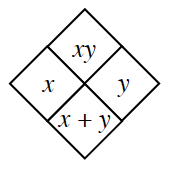1.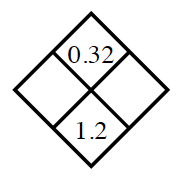What two numbers have a product of $0.32$ and a sum of $1.2$? You may have to use a guess and check method.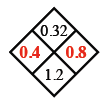1.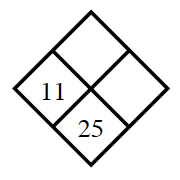$11+?=25$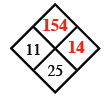1.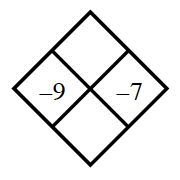What is the product and sum of $-9$ and $-7$?

1.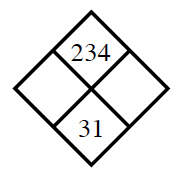See part (a).